Contents

2.4. Metasystem Hierarchies

Consider metasystem hierarchies of computing machines. After we have chosen a universal all-level programming language, such as Refal, a hierarchy of machines becomes a hierarchy of programs. We want to write functions which are defined on definitions of other functions. In every programming language we distinguish objects which are manipulated, from certain special details, variables and function calls, which represent sets of objects and computation processes and cannot be directly treated as objects. Let the set of objects be Sob and the set of variables and function calls Svf. To write a program which manipulates programs, we must map the set of all elements of programs, i.e.,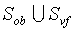, on the set of objects Sob. We call this mapping a metacode, and denote the metacode transformation of e as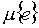: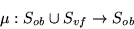Obviously, metacoding must have a unique inverse transformation, demetacoding, so it must be injective: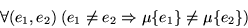For convenience of reading metacoded expressions we require that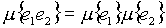. Also, it is desirable that the image of an object expression be as close to the expression itself as possible. It would be nice, of course, to leave all object expressions unaltered under the metacode, but this is, unfortunately, impossible, because it contradicts to the requirement of injectivity. Not every expression e can be represented as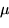{e'}, where e is also an object expression. This creates a hierarchy of MST domains: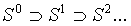where S0 = Sob, and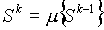, for k > 1. The metacode for Refal which is used in the latest implementation of this language is given in Nemytykh, Pinchuk and Turchin  (see Table 2) in this volume.

Compare two function calls: <F2 <F1 x>> and <F2{<F1 x>}>. The first call is a functional composition: <F1 x> is computed and its value is taken as argument in the computation of F2. In the second call there is no evaluation of <F1 x>, but the metacode of this expression is turned over for the computation of F2. If the metacode of  is used, we have: <F2 ('!'F1('ex'))>. This is an MST hierarchy of two levels: function F2 is supposed to manipulate F1 (its representation, to be precise). If this manipulation is semantic in nature, i.e., based on the definition of F1, as it is in all interesting cases, then the machine F2 must have access to the definition of F1. In order not to encumber our notation, we shall always assume that whenever a metamachine F2 manipulates a machine F1, it incorporates the definition of F1, so we need not indicate this in each case.

We use MST schemes for a clear and metacode-invariant representation of metasystem hierarchies. (I first introduced this notations in my lectures at the University of Copenhagen in 1985. Evere since, its various versions were used in seminars on Refal and metacomputation in Moscow and New York. In a published form it first appeared in .) An MST scheme is built according to the rule: whenever a subexpression has the form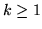, the metacoded part is moved one level down and replaced by dots on the main level: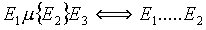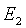This rule can be applied any number of times. To convert an MST scheme into an equivalent Refal expression, we must metacode each level as many times as long is its distance from the top.

MST schemes allow us to represent very clearly certain operations on variables which are necessary for correct construction and use of metasystem hierarchies. I shall show this using as an example the well-known procedure of converting an interpreter for some language into a compiler by partial evaluation (see [9,41,18]).

Let L be an interpreter for some language L written in Refal, and let it be used in the format <L e.prog,e.data>. Let PE be a partial evaluator for Refal written in Refal and having Refal as the target language, i.e., producing a Refal program at the output. We apply PE to the call of L where some program P is substituted for e.prog, while e.data remains free. This call is <L P,e.data>. We metacode it and submit to the partial evaluator:



In this MST scheme the call of L, which is submitted for partial evaluation, is a function of data only, since the value of e.prog is fixed at a specific expression P. After PE performs all operations which can be performed because the program P is known, it outputs a residual program which is nothing else but the translation of the program P into Refal. Function PE has worked as a compiler. Now suppose we want a PE function which would accept an arbitrary program, not just P. If we simply put the variable program instead of P:



we will not get what we want. Here the variables for data and for program are on the same level and are treated in the same way. No partial evaluation takes place, because the value of e.prog remains unknown to PE. Even though e.prog is an argument of L, its value must be provided on the level of PE, so that when L is running (being driven by PE), the program is fixed. We represent this situation by raising e.prog to the top level, and leaving the bullet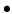in the place where this variable originated on the bottom level:



We shall call the variables like e.prog   elevated. For such a variable, the definition level, at which its name is placed, is different from the usage level indicated by the bullet, and the difference h between the two is the variable's elevation. The value assigned to a variable on the definition level enters the configuration after being metacoded h times. Possible values of an elevated variable belong to the MST domain Sh, and this must be taken into account for correct driving.

The rule of two levels helps read MST schemes: The variables on the top level are free. Those on the next level are bound: they run over their domains as, e.g., integration variables, or the variables in a function definition.

Even though e.prog is used by L, it is not free for it. It is free on the level of the partial evaluation function PE; to run PE we must first substitute some specific program for e.prog. Hence L always receives a fixed program. The result of PE will be a transformed (partially evaluated) function L, which depends only on the variable e.data and is a translation of e.prog from L into Refal. The translation, however, is made directly by PE, which can work with any definition of L (hidden in the function name L, as we agreed above). We can further optimize PE by partially evaluating it by itself according to the MST scheme:



This scheme is a scheme of generation of a compiler from the language L defined by a given interpreter L. Let us read it using the rule of two levels. There are no free variables on the top level: we run the upper PE only once and receive a program which is a function of e.prog: a compiler for L. Now we take one step down and again apply the rule of two levels. The lower PE (the compiler) is on the top. It asks for e.prog on the input and produces a function of e.data as the output. It is the translation of e.prog.

As I mentioned above, one must be aware of the narrowed domains of elevated variables when driving. It is interesting to note, though, that ignoring this leads to actual mistakes only when the MST scheme has at least three levels. In the two-level scheme of compilation above, e.prog is elevated. But it is, at the same time, free. When making a call of PE, we substitute for it a metacoded program (and cannot do otherwise since program is not in Sob); hence the requirement to the value of e.prog becomes satisfied. However, with the third level, the upper PE drives expressions which include unreplaced (free for the lower PE) variable e.prog, and it will consider various contractions for it. If it is not informed that the variable's domain is S1, not the full Sob, it will consider non-existing contractions, which will make the program wrong, and will typically result in an infinite loop.

It often happens that a program transformer must transform a function call which, in fact, can be simply evaluated. The argument may include no free variables or yet uncomputed function calls or, if there are some, they may not be consulted at any stage of evaluation. Even more frequent is a situation where such independence of unknown data holds for a part of the evaluation process, even though not for the whole length of it.

Consider our two-level scheme of compilation. The interpreter L operates on a known program and unknown data. On some stretches of computation L will work on the program, but without consulting the data. An obvious example is the parsing of the program. Further, if the language L includes GO TO statements with jumps to a label, then it may be necessary to examine a big piece of program in search of the needed label. The work of the function PE in this part of computation will be nothing else but simulation of the work of L, which, of course, will take much more time than a direct run of the function L.

In our papers with Nemytykh and, later, Pinchuk [46,25] we describe a supercompiler which makes automatic jumps from one level to another in order to avoid doing on the level n in the interpretation mode what can be done on the level n-1   by direct computation. Before driving a configuration, the supercompiler passes control one level down by demetacoding the configuration and starting the execution of it. If it is possible to bring the computation to the end, the result is metacoded and control returns to the upper level. If at a certain stage of execution its continuation becomes impossible because of unknown values of variables, the configuration of the latest stage preceding the current is metacoded and control passes to the top level for driving.

To make this system work, it was necessary to modify the implementation of Refal by adding a feature which was called a freezer, see . In tests we could see that the use of metasystem jumping can lead to very significant speedups, sometimes by a factor of more than twenty.

Contents  Next